# Get XY Value VI

## LabVIEW 2018 Help

Edition Date: March 2018
Part Number: 371361R-01
View Product InfoLabVIEW 2016 HelpLabVIEW 2017 HelpLabVIEW 2018 HelpLabVIEW 2019 HelpLabVIEW 2020 Help

Owning Palette: Waveform VIs and Functions

Requires: Base Development System

Returns the X and Y value of a waveform or digital data set. The data type you wire to the waveform in input and the data type of the Y component of the analog waveform determine the polymorphic instance to use.

Example

Use the pull-down menu to select an instance of this VI.

 Select an instance WDT Get XY Value DBLWDT Get XY Value I64WDT Get XY Value I32WDT Get XY Value I16WDT Get XY Value CDBWDT Get XY Value EXTWDT Get XY Value CXTDWDT Get XY ValueDTbl Get Digital Value

## WDT Get XY Value DBL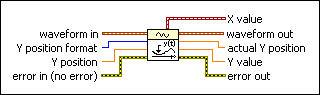waveform in is the analog waveform for which you want to retrieve a specified data value (X and Y value). The default is empty.Y position format indicates if you want to retrieve the data value of the specified element or at a specified time.

 0 Samples—Returns the data value of the specified sample in the waveform data. 1 Relative Time—Returns the data value at a specified time relative to the first point in the waveform.Y position is the element number you want from the set of waveform data or the value at a specified time. The default is 0. For example, if you want the value of the 200th scan, wire 199 to this input. If you want the value at time 100, wire 100 to this input. You select whether this is a samples or relative time Y position format. In Relative Time mode, this VI checks the Y position input to determine if it is an integer multiple of dt. If Y position is not an integer multiple of dt, the VI uses the closest exact multiple of dt.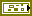error in describes error conditions that occur before this node runs. This input provides standard error in functionality.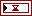X value returns the X value.waveform out returns waveform in unchanged.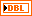actual Y position is the actual index or time value of the point returned.Y value returns the Y value.error out contains error information. This output provides standard error out functionality.

## WDT Get XY Value I64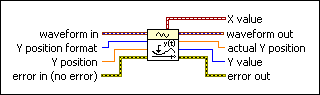waveform in is the analog waveform for which you want to retrieve a specified data value (X and Y value). The default is empty.Y position format indicates if you want to retrieve the data value of the specified element or at a specified time.

 0 Samples—Returns the data value of the specified sample in the waveform data. 1 Relative Time—Returns the data value at a specified time relative to the first point in the waveform.Y position is the element number you want from the set of waveform data or the value at a specified time. The default is 0. For example, if you want the value of the 200th scan, wire 199 to this input. If you want the value at time 100, wire 100 to this input. You select whether this is a samples or relative time Y position format. In Relative Time mode, this VI checks the Y position input to determine if it is an integer multiple of dt. If Y position is not an integer multiple of dt, the VI uses the closest exact multiple of dt.error in describes error conditions that occur before this node runs. This input provides standard error in functionality.X value returns the X value.waveform out returns waveform in unchanged.actual Y position is the actual index or time value of the point returned.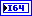Y value returns the Y value.error out contains error information. This output provides standard error out functionality.

## WDT Get XY Value I32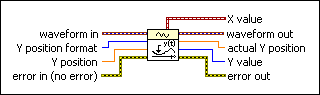waveform in is the analog waveform for which you want to retrieve a specified data value (X and Y value). The default is empty.Y position format indicates if you want to retrieve the data value of the specified element or at a specified time.

 0 Samples—Returns the data value of the specified sample in the waveform data. 1 Relative Time—Returns the data value at a specified time relative to the first point in the waveform.Y position is the element number you want from the set of waveform data or the value at a specified time. The default is 0. For example, if you want the value of the 200th scan, wire 199 to this input. If you want the value at time 100, wire 100 to this input. You select whether this is a samples or relative time Y position format. In Relative Time mode, this VI checks the Y position input to determine if it is an integer multiple of dt. If Y position is not an integer multiple of dt, the VI uses the closest exact multiple of dt.error in describes error conditions that occur before this node runs. This input provides standard error in functionality.X value returns the X value.waveform out returns waveform in unchanged.actual Y position is the actual index or time value of the point returned.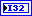Y value returns the Y value.error out contains error information. This output provides standard error out functionality.

## WDT Get XY Value I16waveform in is the analog waveform for which you want to retrieve a specified data value (X and Y value). The default is empty.Y position format indicates if you want to retrieve the data value of the specified element or at a specified time.

 0 Samples—Returns the data value of the specified sample in the waveform data. 1 Relative Time—Returns the data value at a specified time relative to the first point in the waveform.Y position is the element number you want from the set of waveform data or the value at a specified time. The default is 0. For example, if you want the value of the 200th scan, wire 199 to this input. If you want the value at time 100, wire 100 to this input. You select whether this is a samples or relative time Y position format. In Relative Time mode, this VI checks the Y position input to determine if it is an integer multiple of dt. If Y position is not an integer multiple of dt, the VI uses the closest exact multiple of dt.error in describes error conditions that occur before this node runs. This input provides standard error in functionality.X value returns the X value.waveform out returns waveform in unchanged.actual Y position is the actual index or time value of the point returned.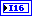Y value returns the Y value.error out contains error information. This output provides standard error out functionality.

## WDT Get XY Value CDB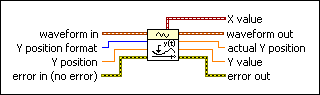waveform in is the analog waveform for which you want to retrieve a specified data value (X and Y value). The default is empty.Y position format indicates if you want to retrieve the data value of the specified element or at a specified time.

 0 Samples—Returns the data value of the specified sample in the waveform data. 1 Relative Time—Returns the data value at a specified time relative to the first point in the waveform.Y position is the element number you want from the set of waveform data or the value at a specified time. The default is 0. For example, if you want the value of the 200th scan, wire 199 to this input. If you want the value at time 100, wire 100 to this input. You select whether this is a samples or relative time Y position format. In Relative Time mode, this VI checks the Y position input to determine if it is an integer multiple of dt. If Y position is not an integer multiple of dt, the VI uses the closest exact multiple of dt.error in describes error conditions that occur before this node runs. This input provides standard error in functionality.X value returns the X value.waveform out returns waveform in unchanged.actual Y position is the actual index or time value of the point returned.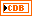Y value returns the Y value.error out contains error information. This output provides standard error out functionality.

## WDT Get XY Value EXT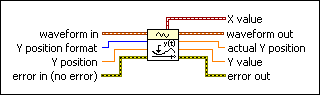waveform in is the analog waveform for which you want to retrieve a specified data value (X and Y value). The default is empty.Y position format indicates if you want to retrieve the data value of the specified element or at a specified time.

 0 Samples—Returns the data value of the specified sample in the waveform data. 1 Relative Time—Returns the data value at a specified time relative to the first point in the waveform.Y position is the element number you want from the set of waveform data or the value at a specified time. The default is 0. For example, if you want the value of the 200th scan, wire 199 to this input. If you want the value at time 100, wire 100 to this input. You select whether this is a samples or relative time Y position format. In Relative Time mode, this VI checks the Y position input to determine if it is an integer multiple of dt. If Y position is not an integer multiple of dt, the VI uses the closest exact multiple of dt.error in describes error conditions that occur before this node runs. This input provides standard error in functionality.X value returns the X value.waveform out returns waveform in unchanged.actual Y position is the actual index or time value of the point returned.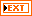Y value returns the Y value.error out contains error information. This output provides standard error out functionality.

## WDT Get XY Value CXTwaveform in is the analog waveform for which you want to retrieve a specified data value (X and Y value). The default is empty.Y position format indicates if you want to retrieve the data value of the specified element or at a specified time.

 0 Samples—Returns the data value of the specified sample in the waveform data. 1 Relative Time—Returns the data value at a specified time relative to the first point in the waveform.Y position is the element number you want from the set of waveform data or the value at a specified time. The default is 0. For example, if you want the value of the 200th scan, wire 199 to this input. If you want the value at time 100, wire 100 to this input. You select whether this is a samples or relative time Y position format. In Relative Time mode, this VI checks the Y position input to determine if it is an integer multiple of dt. If Y position is not an integer multiple of dt, the VI uses the closest exact multiple of dt.error in describes error conditions that occur before this node runs. This input provides standard error in functionality.X value returns the X value.waveform out returns waveform in unchanged.actual Y position is the actual index or time value of the point returned.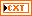Y value returns the Y value.error out contains error information. This output provides standard error out functionality.

## DWDT Get XY Value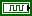waveform in is the digital waveform for which you want to retrieve a specified data value (X and Y value). The default is empty.Y position format indicates if you want to retrieve the data value of the specified element or at a specified time.

 0 Samples—Returns the data value of the specified sample in the waveform data. 1 Relative Time—Returns the data value at a specified time relative to the first point in the waveform.Y position is the element number you want from the set of waveform data or the value at a specified time. The default is 0. For example, if you want the value of the 200th scan, wire 199 to this input. If you want the value at time 100, wire 100 to this input. You select whether this is a samples or relative time Y position format. In Relative Time mode, this VI checks the Y position input to determine if it is an integer multiple of dt. If Y position is not an integer multiple of dt, the VI uses the closest exact multiple of dt.error in describes error conditions that occur before this node runs. This input provides standard error in functionality.X value returns the X value.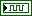waveform out returns waveform in unchanged.actual Y position is the actual index or time value of the point returned.Y value returns an array of Y values.error out contains error information. This output provides standard error out functionality.waveform data value returns the values of the waveform data.

## DTbl Get Digital Value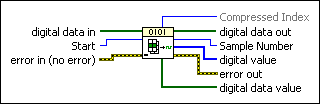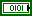digital data in is the set of digital data for which you want to retrieve the X and Y values.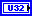Start is the data element or time value where you want to start acquiring X and Y values.error in describes error conditions that occur before this node runs. This input provides standard error in functionality.Compressed Index returns the index of the data element or time value with respect to the compressed waveform. For example, if the actual sample number is 8 but the sample is compressed in a portion of the waveform that starts at 3, the compressed index is 3.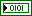digital data out returns digital data in unchanged.Sample Number returns the sample number of the data element or time value. If the value of Start is inside a compressed portion of the waveform, Sample Number is the sample number calculated as if the waveform was not compressed.digital value returns an array of Y values.error out contains error information. This output provides standard error out functionality.digital data value returns the values of the digital data.

## Example

Refer to the Waveform - Get Y Value VI in the labview\examples\Waveform directory for an example of using the Get XY Value VI.